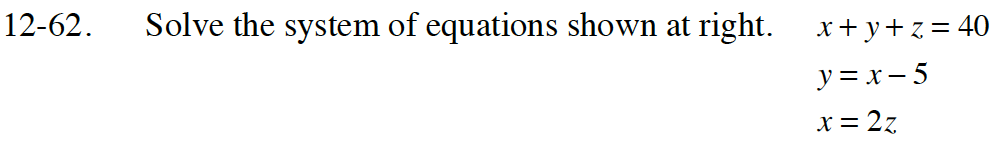### Home > INT3 > Chapter 12 > Lesson 12.1.4 > Problem12-62

12-62.
1. Solve the system of equations below. Homework Help ✎

2. x + y + z = 40
y = x – 5
x = 2zUse substitution to solve.

If x = 2z, then y = 2z − 5.

Now that you have expressions for both x and y in terms of z, substitute them into the first equation and solve for z.
Then solve for x and y.